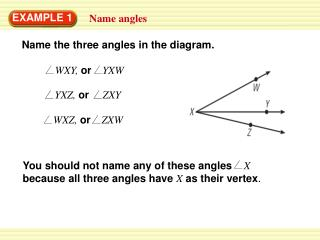# EXAMPLE 1 - PowerPoint PPT PresentationDownload PresentationEXAMPLE 1

EXAMPLE 1
Download Presentation## EXAMPLE 1

- - - - - - - - - - - - - - - - - - - - - - - - - - - E N D - - - - - - - - - - - - - - - - - - - - - - - - - - -
##### Presentation Transcript

1. Name the three angles in the diagram. WXY, or YXW YXZ, or ZXY WXZ, or ZXW You should not name any of these angles X because all three angles have Xas their vertex. EXAMPLE 1 Name angles

2. Use the diagram to find the measure of the indicated angle. Then classify the angle. a.KHJ b.GHK c.GHJ d.GHL EXAMPLE 2 Measure and classify angles SOLUTION A protractor has an inner and an outer scale. When you measure an angle, check to see which scale to use.

3. o a. HJ is lined up with the 0 on the inner scale of the protractor. HKpasses through 55 on the inner scale. So, mKHJ = 55 . It is an acute angle. o o o b. HG is lined up with the 0 on the outer scale and HKpasses through 125 on the outer scale. So, mGHK = 125 . It is an obtuse angle. o o o c. m GHJ = 180. It is a straight angle. o d. m GHL= 90. It is a right angle. EXAMPLE 2 Measure and classify angles

4. 1. Name all the angles in the diagram. Which angle is a right angle? ANSWER PQR , PQS, RQS ; PQSis a right angle . for Examples 1and 2 GUIDED PRACTICE

5. ANSWER Straight Angle for Examples 1and 2 GUIDED PRACTICE 2. Draw a pair of opposite rays. What type of angle do the rays form?